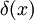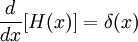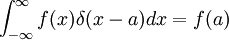# Dirac delta distribution

The Dirac delta distribution (or generalized function) is written as$\delta(x)$. It is the derivative of the Heaviside step distribution,$\frac{d}{dx}[H(x)] = \delta(x)$$\int_{- \infty}^{\infty} f(x) \delta (x-a) dx = f(a)$